×#### Thank you for registering.

One of our academic counsellors will contact you within 1 working day.

Click to Chat

1800-1023-196

+91-120-4616500

CART 0

• 0

MY CART (5)

Use Coupon: CART20 and get 20% off on all online Study Material

ITEM
DETAILS
MRP
DISCOUNT
FINAL PRICE
Total Price: Rs.

There are no items in this cart.
Continue Shopping• Complete JEE Main/Advanced Course and Test Series
• OFFERED PRICE: Rs. 15,900
• View Details

```Chapter 3: Decimals Exercise – 3.1

Question: 1

Write each of the following as decimals:

(i)  8/100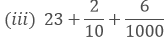Solution:

(i)  8/100

Mark the decimal point two places from right to left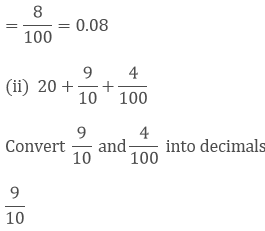Mark the decimal point one place from right to left

9/10 = 0.9

4/100

Mark the decimal point two places from right to left

4/100

= 0.04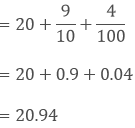Convert 2/10 and 6/1000 into decimals

2/10

Mark the decimal point one place from right to left

2/10

= 0.2

6/1000

Mark the decimal point three places from right to left

6/1000

= 0.006

23, 0.2, 0.006 are unlike decimals. So we convert them into like decimals.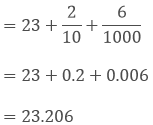Question: 2

Convert each of the following into fractions in the lowest form:

(i) 0.04

(ii) 2.34

(iii) 0.342

(iv) 17.38

Solution:

(i) 0.04

= 0.04/1= 4/100

= 1/25

(ii) 2.34

= 2.34/1= 234/100

= 117/50

(iii) 0.342

= 0.342/1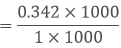= 342/1000

= 171/500

(iv) 17.38

=17.38/1= 1738/100

= 869/50

Question: 3

Express the following fractions as decimals:

(i)  23/10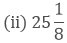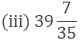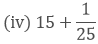Solution:

(i)  23/10

= 23/10

= 2.3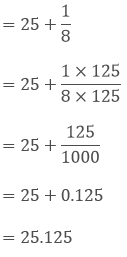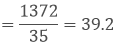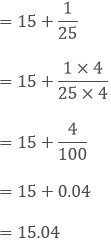Question: 4

(i) 41.8, 39.24, 5.01 and 62.6

(ii) 18.03, 146.3, 0.829 and 5.324

Solution:

(i) 148.65

(ii) 170.483

Question: 5

Find the value of:

(i) 9.756 - 6.28

(ii) 48.1- 0.37

(iii) 108.032 - 86.8

(iv) 100 - 26.32

Solution:

(i) 3.476

(ii) 47.73

(iii) 21.232

(iv) 73.68

Question: 6

Take out 3.547 from 7.2

Solution:

3.653

Question: 7

What is to be added to 36.85 to get 59.41?

Solution:

x + 36.85 = 59.41

x = 59.41-36.85

x = 22.56

Therefore 22.56 is added to 36.85 to get 59.41

Question: 8

What is to be subtracted from 17.1 to get 2.051?

Solution:

17.1- x = 2.051

17.1 = x + 2.051

x = 17.1 - 2.051

x = 15.049

Therefore 15.049 is subtracted from 17.1 to get 15.049

Question: 9

By how much should 34.79 be increased to get 70.15?

Solution:

34.79 + x = 70.15

x = 70.15 - 34.79

x = 35.36

Therefore 35.36 is increased to 70.15

Question: 10

By how much should 59.71 be decreased to get 34.58?

Solution:

59.71 – x = 34.58

59.71 - 34.58 = x

x = 25.13

Therefore 25.13 is decreased to get 34.58
```### Course Features

• 728 Video Lectures
• Revision Notes
• Previous Year Papers
• Mind Map
• Study Planner
• NCERT Solutions
• Discussion Forum
• Test paper with Video Solution